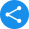# NCERT Solutions for Class 5 Maths

## Maths Class 5 NCERT Solutions

Mathematics is a subject which incites fear in the heart of most students. They mainly face problems with the subject because they do not have a clear understanding of its basics. However, now you can make understanding maths easier by referring to our latest NCERT solutions Class 5 Maths. It provides a comprehensive solution for every problem in your textbook.

The CBSE NCERT solutions contain the answers to the exercises from the NCERT Maths book, which are crucial for your examinations. These chapters also help to prepare you for the higher classes making these NCERT solutions download even more important.

Our expert teachers approach each question in a unique way. Through interesting pictures and 3D models, we provide a brand new twist to the textbook solutions. We also try to make CBSE Board mathematics syllabus comparatively easier with our NCERT solutions online. At the end of each chapter, there are several fun activities and exercises which help you to reach your full potential. If you follow the exercises regularly, you are bound to score high in your maths exams. You can download our NCERT solutions PDF from anywhere anytime, absolutely free of cost.

The syllabus for NCERT Class 5 Maths consists of 14 chapters which are widely different from one another. They provide students with a strong foundation for the upcoming years and NCERT textbook solution for Class 5 Maths explains each problem very accurately.

The following are the chapters included in the solutions as per the latest syllabus, which will help you grasp the basic concepts:

Chapter 1: The Fish Tale

This chapter teaches students about multiplications through engaging illustrations like fishes and boats. Students will learn the concept of weight, distance, and money from this context. You can clarify your doubts regarding this chapter through our NCERT solutions download.

Chapter 2: Shapes and Angles

The chapter provides the concept of shapes and angles. The NCERT textbook solution for Class 5 Maths includes a solution to the problems through colourful pictures, audio, video, and 3D models. This innovative and interactive way surely intrigues a child’s curious mind.

Chapter 3: How many squares?

You will learn how to divide into equal squares. Latest NCERT solutions Class 5 Maths also teach how to measure a square. Solve the exercises provided at the end to get a clear concept of the chapter.

Chapter 4: Parts and Wholes

You will get to learn about parts of different shapes and areas through this chapter. Latest NCERT solutions Class 5 Maths provides a fun solution to these problems. You will also get to calculate the area of a flag or any other object.

Chapter 5: Does it look the same?

Through this chapter, you will get to know what are the different categories of shapes and how can they be compared to each other. In the free NCERT solution for Class 5 Maths, we solve the problems of this chapter through colourful pictures and 3d models.

Chapter 6: Be my multiple, I’ll be your factor

This chapter introduces you with the concept of least common multiple, which means students get to learn how to find the lowest multiple of a series of numbers. NCERT Class 5 Maths solutions to each question in the textbook are provided by us to clear all your doubts.

Chapter 7: Can you see the pattern?

This chapter will educate you about different shapes and how you can change the shapes by changing their directions. You can find the latest NCERT solutions Class 5 Maths to the problems of the Class 5 mathematics textbook here.

Chapter 8: Mapping your way?

You will know how to calculate distances between two points. You will also learn why an object appears smaller when the distance between you and the object increases. After going through the chapter, refer to the Class 5 Maths NCERT book solutions to understand it better.

Chapter 9: Boxes and Sketches

In this chapter, you will learn how one box can create several different shapes. We provide Class 5 Maths NCERT solutions to these problems using 3D images, models, and animation.

Chapter 10: Tenths and Hundredths

Through this chapter, you will get to learn how to use a ruler to measure anything in centimeters. You will also learn to measure height and tell which object is taller among two given objects. The latest NCERT solutions Class 5 Maths will clear your doubts regarding this chapter.

Chapter 11: Area and its boundary

This chapter will teach you how to calculate areas of squares and rectangles; what is the difference between area and length. You can then refer to the CBSE NCERT solutions Class 5 Maths to learn how to apply the calculations to solve problems.

Chapter 12: Smart charts

You will learn about charts and diagrams through this chapter. We provide the latest NCERT solutions Class 5 Maths to the questions regarding charts, graphs, and pie charts.

Chapter 13: Ways to multiply and divide

This chapter is one of the most crucial ones in the syllabus since it introduces you to the idea of multiplications and divisions, which are essential for your higher studies. We try to teach you this chapter through fun activities and secure learning method. You can get a better idea about this topic if you view our NCERT solutions online.

Chapter 14: How big? How heavy?

You will be introduced to the various units of measurement through this chapter. You will get to know how you can measure the weight of different objects accurately. NCERT solutions PDF provides an exciting approach to the measurements.

### NCERT Solutions for Class 5 Maths

Share this with your friendsShare